# 6 Formulas and Functions

• Types of functions and the syntax of a function.
• Entering a function, via the Wizard and manually.
• Introduction to
• math functions
• statistical functions
• date/time functions
• logic functions
• search functions
• Nesting of functions.

Excel has more than 300 built-in functions to perform a variety of operations. Features of the functions are divided into several categories, such as

• Database
• Date & Time
• Financial
• Information
• Logical
• Statistical
• Engineering
• Text
• Math & Trig
• Lookup & Reference

A category can be useful when you are looking for a particular function. For example, if you are looking for a function to calculate the repayment of a loan, then this function can be found in the Financial category. Also, there are other categories: All, Most recently used and User defined.

With most of the features you need to specify what values or contents of cells the function should use in the calculation. These entries are called arguments.

All functions in Excel have the same construction (syntax), see figure 6.1.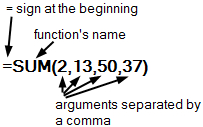Figure 6.1: The structure (syntax) of a function.

• They start with the equal sign =.
• Then the function’s name.
• Then round brackets (parentheses) enclosing the arguments. The arguments are separated by the so-called argument separator the comma (,).
• The arguments may include numbers, texts, operations, or also other functions.
1. The separator for the arguments depends on a Windows setting. Default is the comma for English versions and a semicolon for the Dutch versions. You can change this through the control panel of Windows.

2. The function’s name and the separation of arguments depends on the used language version in Microsoft Office.

Some commonly used functions are discussed through tasks in this chapter.

## 6.1 Entering Functions

You can enter a function via a Wizard, but you can also enter it manually. Which method is best to use mainly depends on how experienced you are and whether you already know the name of the position and its structure. Both methods are explained below. The Wizard is used for the tasks in this chapter, but you can of course also use the manual method.

### Insert function with the Wizard

Beginners and less experienced users should use the Function Wizard. You can call this Wizard in two ways

• By clicking on the button $$f_x$$ Insert function at the beginning of the formula bar.
• Through Formulas > Insert Function (group Function Library)

In both cases the dialog box Insert Function appears: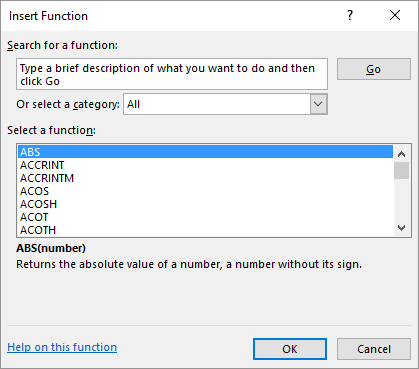Figure 6.2: Dialog box insert function.

To quickly find the right function, it is useful to first select a category of functions by clicking the selection arrow in the category box. The categories appear in the drop-down window. If you have no idea in which category the function belongs, then choose All.

Select the desired function, for example COUNTIF and click on OK. After this, the Function Arguments dialog box for the selected function appears.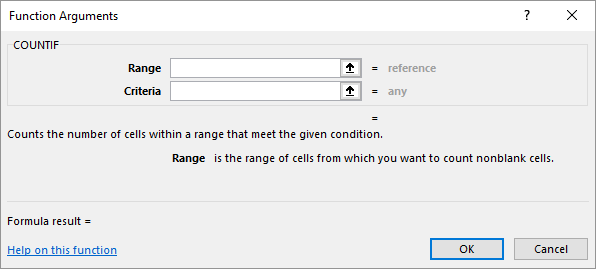Figure 6.3: Function arguments for function COUNTIF.

You can specify the arguments in the dialog box. Which argument(s) you should use depends on the selected function. You can type in the arguments, but when it comes to cell addresses you can also select these cells in the worksheet with the mouse.

By clicking the link Help on this function you get detailed help for the function.

### Functie intypen met automatisch aanvullen

When you are familiar with a particular function, knowing the correct spelling and the types of arguments, you can choose to just type the function and its arguments into the input bar. Often this method is the most efficient.

A very useful tool for this is Autocomplete functions. When you type a = character and the first letter of a function in a cell or in the input bar, Excel displays a drop-down list of all valid entries that start with that letter. Icons show the type of entry, such as a function or cell/table reference. And for each function you get a screen tip with a short description of the function.

You can continue typing the function to narrow the list or use the arrow keys to select the function from the list. After selecting the desired function, press Tab to insert the function and the opening parenthesis into the cell.

After you press Tab to insert the function and the opening parenthesis, Excel displays another screen tip with the arguments for the function. The argument in bold is the argument you are currently entering. Arguments in parentheses are optional.

In figure 6.4 you can see an example. In the first image, one letter, the c, has been entered. The list box shows all functions that start with c.

In the second image another two letters ‘ou’ has been typed. The list now consists of all items that start with cou. With the selected function ‘COUNTIF’ a screen tip is shown.

After pressing Tab you get the third image. This function has two arguments.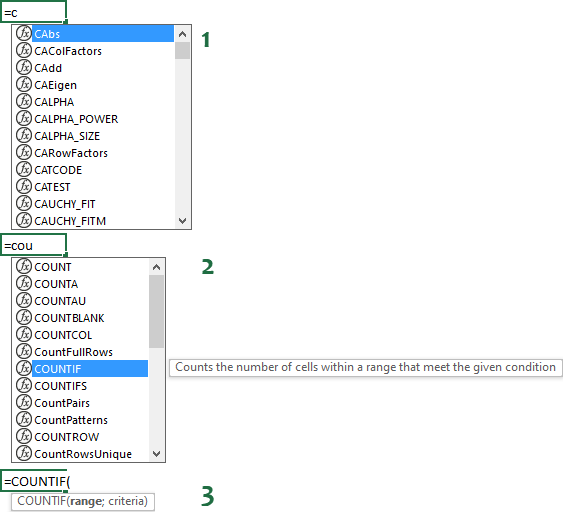Figure 6.4: Example autocomplete function.

Entering a function via the Wizard often requires several mouse clicks. Because addition is the most used operation, Excel has a separate button, called Autosum, on the ribbon in the Editing group (Home tab) so that you can enter this function faster.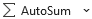Through the arrowhead at the end you can also get a selection list for the functions Average, Count Numbers, Max and Min, so these functions are also quickly accessible.

2. Enter in the cells A1:A6 in succession the following numbers: 120, 121, 122, 123, 124, and 125.

3. Select cell A7 and then click on button Autosum.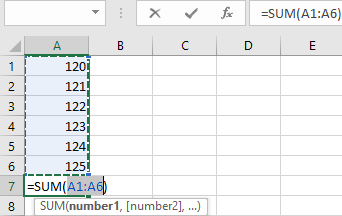Figure 6.5: Selection borders around cells to sum.

Excel places a selection border around the cells that you probably want to sum. If this is not the area you want to sum, then select with the mouse the correct area.

1. Confirm the selection by one of the following actions:

• Click again on the Autosum button.
• Click on buttonEnter in front of of the formula bar.
• Press the Enter key on the keyboard.

In cell A7 the answer 735 is displayed. The formula in cell A7 is =SUM(A1:A6). This formula is shorter and clearer, therefore better than a formula like =A1+A2+A3+A4+A5+A6.

The mathematical functions category is quite extensive. Besides the well-known SUM function for adding numbers, this category contains functions for various computing activities, such as exponentiation, root calculations, PI, logarithms, etc. Furthermore, you will find a lot of trigonometric functions. So all kinds of functions for a specific use.

More common functions are those for rounding numbers. Excel has thirteen functions for rounding and that is why it can be quite confusing to determine which function to use in a given situation. For example, there is a function ROUND for rounding a number up or down to a specified number of decimal places. There are also functions for rounding to the nearest multiples of a given number or rounding to an integer value.

Figure 6.6 shows the results of a number of rounding functions. Column C indicates which formulas have been used for column B.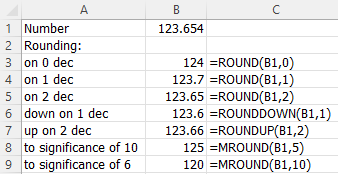Figure 6.6: Examples of rounding functions.

File: Rounding.xlsx

1. Open the practice file.

2. Enter the appropriate formulas in cells B3:B9 using the Function Wizard.

The statistical category contains a variety of functions for statistical analysis like average, count, minimum, and maximum.

File: Statistics.xlsx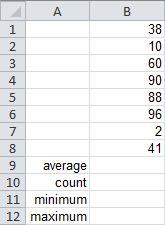Figure 6.7: File Statistics.xlsx to practice statistical functions.

1. Open the practice file.

2. In cells B9 to B12, perform the calculations using the functions from the table below. The functions are in the category Statistical.

cel functie
B9 AVERAGE
B10 COUNT
B11 MIN
B12 MAX

The argument is always the area B1:B8. You can of course type this in the function arguments dialog box, but it is more convenient to place the cursor in the box for the first argument and then select the area B1:B8 in the worksheet with the mouse.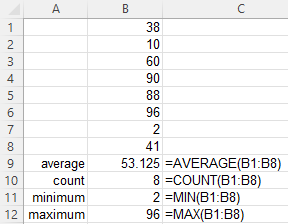## 6.5 Task: Date and Time Functions

In the Date and Time category, you will find multiple functions to perform calculations with date and time. Because Excel sees date and time internally as numbers you can just calculate with date and time. American date format: Month/Day/Year

File: Date.xlsx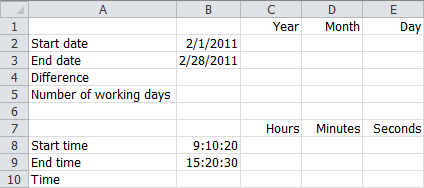Figure 6.8: Calculation with dates and times..

1. Open the practice file.

First of all, the values for year, month and day are determined from the start and end dates.

1. Use the YEAR, MONTH and DAY functions to determine the values in cells C2, D2, and E2. The argument is always cell B2.

2. Select C2:E2 and drag the fill handle 1 row down.

3. Select cell B4 and enter the formula =B3-B2. The result will be 27.

With the function NETWORKDAYS you can determine the number of full working days between a start date and an end date. Weekends and dates defined as holidays do not count as working days. This function contains an optional argument to specify holidays.

1. Select cell B5 and calculate the number of working days. You only need to enter the cell addresses for start date and end date, you can leave the box for holidays empty.

2. The same way calculate the hours, minutes, and seconds from the start date and end date. Use the functions HOUR, MINUTE, and SECOND.

3. Calculate in B10 the time difference with a formula.

You have to subtract the start time from the end time.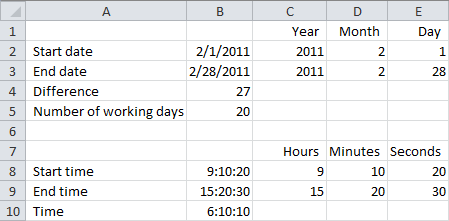## 6.6 Task: Logical Function IF

Logical functions do something with the results TRUE or FALSE. The best known and most widely used logical function is the IF() function. Simply said, the operation of this function does the following:

=IF(condition, what to do if true, what to do if false)

The condition is a logical test with possible results TRUE or FALSE.

If you need more than one condition, then you can use a new IF() function inside the first IF() function. Such structures become quickly complicated and difficult to read. Better make use of the logical functions AND(), OR(), and NOT().

In figure 6.9 you see some marks, with next to it the statement pass or fail. This result should be automatically determined from the value of the grade. A result is unsatisfactory if the number is less than 5.5.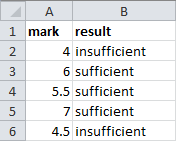Figure 6.9: marks with their results.

1. Start with a new worksheet and enter the numbers as shown in figure 6.9.

2. Select cell B2 and choose via Insert Function the function IF.

3. Complete the dialog box as shown in figure 6.10. You can enter the quotation marks around the texts, but you don’t have to, Excel will place them automatically.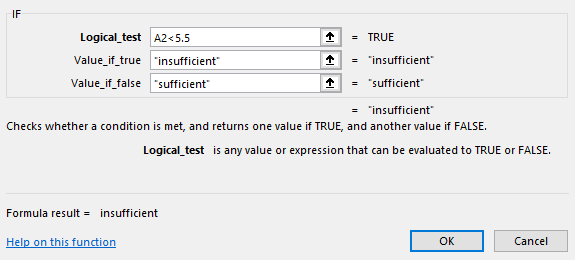Figure 6.10: Dialog box function IF.

1. Click OK.

2. Select cell B2 and drag the fill handle to B6.

A task splitting a text into different parts by using four text functions.

Excel has several functions for performing operations on texts. For example, you can determine the length of a text and retrieve certain pieces of text from a larger text.

In figure 6.11, you see some full names in column A. The idea is to extract the first and last names out of the full names and put these in separate columns. In the example, the first and last names are separated by a space. This makes it possible to split the text at this point. To extract the different parts you need to know the location of the space. The text before the space is the first name and the text after the space is the last name. To determine the right part, you also need to know the total length of the text.

The result of the operations can be seen in figure 6.11 in columns B to E. Because 4 different functions are used, the practice is set up in four parts.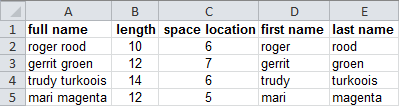Figure 6.11: Full names and the extracted fiirst and last names.

#### LEN

Determination of the length of a text with function LEN.

File: Names.xlsx

1. Open the practice file.

2. Select cell B2 and insert function LEN with argument A2.

3. Drag the fill handle in B2 down to B5.

You now have the length of the full names in column B..

#### LEFT

The left part with a certain length of a text can be determined with the function LEFT. In this example, the first name is the left part.

1. Select cell D2, insert the function LEFT and specify the arguments as shown in figure 6.13.

In the Num-chars box, you need to specify the length of the text to extract. This is one less than the position of the space and that position can be found in cell C2.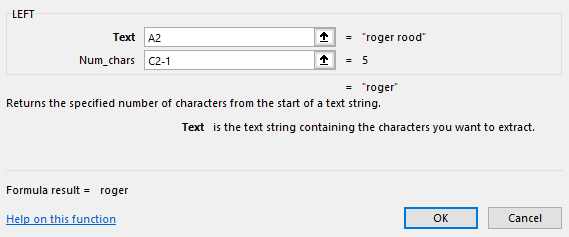Figure 6.13: Arguments for function LEFT.

1. Click OK.

2. Drag the fill handle in D2 down to D5.

The first names are now in column D.

#### RIGHT

The right part with a certain length can be determined with the function RIGHT. In this example, the last name is the right part.

1. Select cell E2, insert the function RIGHT and specify the arguments as shown in figure 6.14.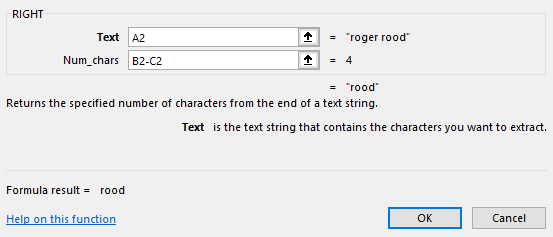Figure 6.14: Arguments for function RIGHT.

1. Click OK.

2. Drag the fill handle in E2 down to E5.

The last names are now in column E.

## 6.8 Task: Entering Nested Functions

A function as an argument inside another function is a nested function. Inside the parenthesis of a function, there is another function. An example:

=ROUNDDOWN(SUM(A1:A5),0)

This nested function will be used in the following task.

1. Start with a new worksheet and enter the data you can see in figure 6.15.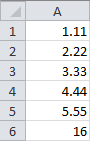Figure 6.15: Data to use with nested function.

1. Select cell A6 and insert function ROUNDDOWN (category Math & Trig).

In the dialog box for this function’s arguments, the cursor is in the Number box. It is handy to first specify the number of decimal places and after that the range of numbers.

1. Put the cursor in the Num_digits box and enter 0, and put the cursor back in Number box.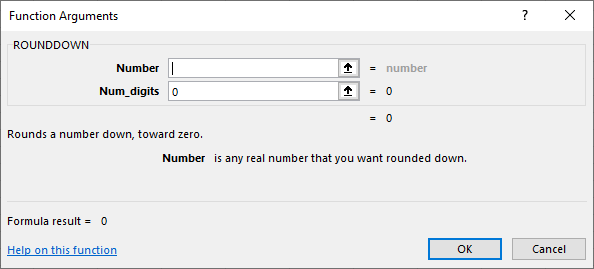Figure 6.16: Dialoogvenster.

1. Click now in the worksheet on the arrow in the name box.

A drop-down list with the names of the most recently used functions appears and at the bottom of the list More functions.

1. Click on More functions. The well known Insert Function dialog box appears.

2. Select in the Math & Trig category the function SUM and click OK.

The Function Arguments dialog box appears. Argument Number1 has already the value A1:A5.

1. Click OK.

The answer now appears in cell A6 and the used formula is displayed in the formula bar.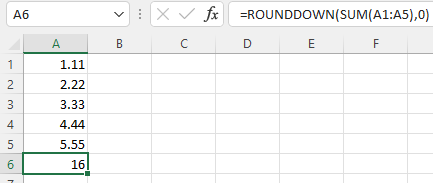Figure 6.17: Result nested function.

1. If you had not entered the number of decimals after step 3, you would have received a warning during nesting that too few arguments were entered. You would have had to correct this manually in the formula.

2. A faster way of working is to manually type the formula in the formula bar. While you are typing, you will receive help with entering. Handling this way, you must know the names of the functions to be used.

The COUNTIF function is very useful when creating frequency tables. In figure 6.18, you see a list of camping sites with their category. In column E should come the number of campsites in each category.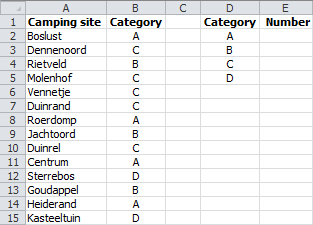Figure 6.18: Campsites by category.

File: Campsites.xlsx

1. Open the practice file.

2. Select cell E2, insert the function COUNTIF and specify the arguments as shown in figure 6.19.Figure 6.19: Arguments for the function COUNTIF.

By specifying the entire column B as range, new rows with data can be added to the bottom without the need to change the formulas.

1. Click OK.

2. Select cell E2 and drag the fill handle down to E5.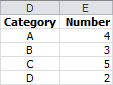The SUMIF function can be used to add numbers under certain conditions. The spreadsheet has a list of the yields of different coffee types per month and per region. You need to determine the total yields per region.

File: Coffee.xlsx

1. Open the practice file.

2. Select cell H2, insert the function SUMIF and specify the arguments as shown in figure 6.20.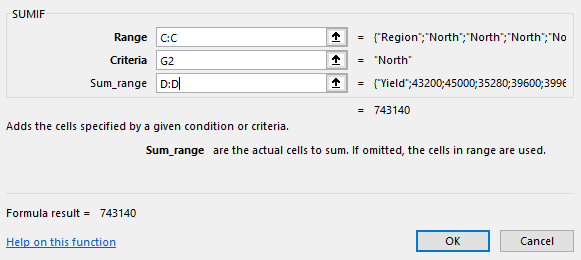Figure 6.20: Arguments for function SUMIF.

1. Click OK. The total yield for region North 743140 now appears in cell H2.

2. Select cell H2 and drag down the fill handle to H5.

3. Format the numbers in H2:H5 as currencies.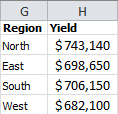An addition in this example is easier to create with pivots.

There are several ways to determine the repayment of a loan. The following example calculates the size of the monthly repayment of a loan of $250,000 with a 6% fixed annual interest rate over a period of 15 years according to the annuity. For this, the Excel function PMT will be used. This function calculates the payment for a loan based on constant periodic payments and a constant interest rate.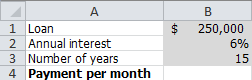Figure 6.21: Data to use for loan repayment. File: Repayment.xlsx 1. Open the practice file and format the cells as shown in figure 6.21. 2. Select cell B4, insert the function PMT (category Financial) and specify the arguments as shown in figure 6.22.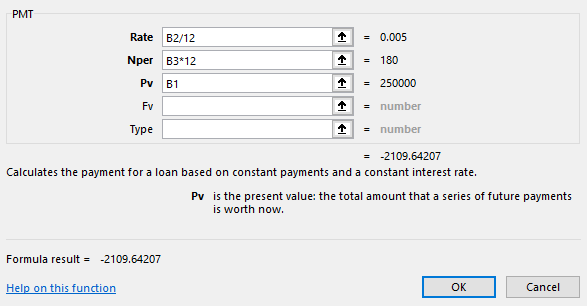Figure 6.22: Arguments for function PMT. Because the period in this example is in months and not in years, the annual rate must be divided by 12 and the number of years must be multiplied by 12. 1. Click OK. The answer -2109.64 appears. Because the result is an amount to be paid, so a debt, Excel displays this as a negative number. That is, in a red font and with a minus sign. 1. Change this in a positive number by putting a minus sign after the = sign. So the formula becomes =-PMT(B2/12,B3*12,B1) ## 6.12 Task: Calculating Number of Periods When taking a personal loan of € 4000 you agreed to pay a monthly amount of € 125. Calculate the number of months you have to repay at a 6% fixed annual interest rate. For this calculation, the Excel function NPER will be used. This function calculates the number of periods for an investment based on periodic, constant payments and a constant interest rate. Payments are entered as negative numbers.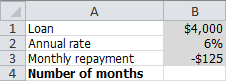Figure 6.23: Calculation number of periods. File: Payment_Periods.xlsx 1. Open the practice file. 2. Select cell B4, insert the function NPER(category Financial) and specify the arguments as shown in figure 6.24.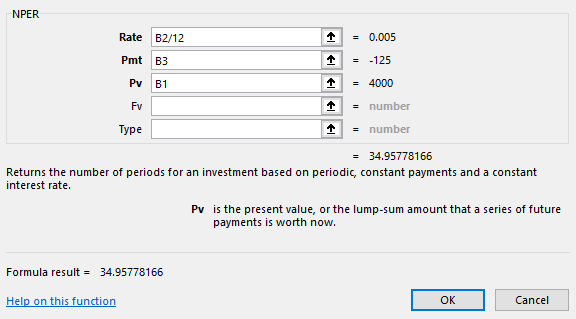Figure 6.24: Arguments for function NPER. Because the period is in months and not in years, the annual rate has been divided by 12. 1. Click OK. The answer 34,95778166 appears, so almost 35 months. ## 6.13 Task: Vertical Lookup The VLOOKUP function looks up for a supplied value in the first column of a list (table) and returns the corresponding value from another column. Syntax: VLOOKUP(lookup-value, table-array, col-index-num, [range-lookup]) The last argument is optional and can be set to FALSE or TRUE. • FALSE: Search for an exact match with the supplied lookup-value. • TRUE: Give the closest match below the supplied value if an exact match can not be found. Most of the time it is best to search for an exact match, otherwise incorrect results are possible. If an approximation is allowed then the left column of the table-array must be in ascending order. In figure 6.25 is an overview of hard drives, with the article number in the first column. With the VLOOKUP function, you can find the capacity, type, and price of an article if you specify the article number.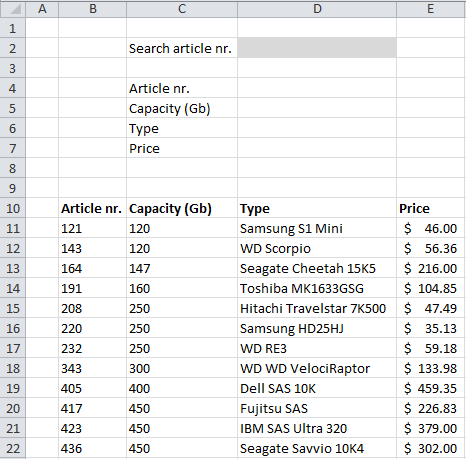Figure 6.25: Overview hard drives. Cell D2 is the input cell for the article number to be searched. The cells D4:D7 are for the lookup formulas. File: Harddisks.xlsx 1. Open the practice file. 2. Select cell D4 and enter the formula: =VLOOKUP($D$$2,$$B$$11:$$E$22,1,FALSE). As result, the error #N/A appears in the cell. This is because there is no search value in cell D2. 1. Enter in cell D2 the value 208. In cell D4 now appears 208, the article number. 2. Now enter the correct lookup formulas in the other three cells. • in D5 the formula: =VLOOKUP($D$$2,$$B$$11:$$E$22,2,FALSE). • in D6 the formula: =VLOOKUP($D$$2,$$B$$11:$$E$22,3,FALSE). • in D7 the formula: =VLOOKUP($D$$2,$$B$$11:$$E$22,4,FALSE).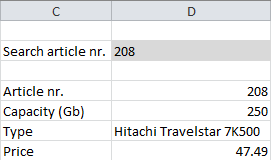Figure 6.26: Results VLOOKUP. ## 6.14 Task: Horizontal Lookup The HLOOKUP function looks up for a supplied value in the first row of a list (table) and returns the corresponding value from another row. Syntax: VLOOKUP(lookup-value, table-array, row-index-num, [range-lookup]) The last argument is optional and can be set to FALSE or TRUE. • FALSE: Search for an exact match with the supplied lookup-value. • TRUE: Give the closest match below the supplied value if an exact match can not be found. It is mostly the best to specify to search for an exact match, otherwise incorrect results are possible. And if an approximation is allowed then the first row of the table-array must be in ascending order. In figure 6.27 is an overview of shoe sizes for some people with the name in the first row. When you specify the name you can find the corresponding age, length, and shoe size with the HLOOKUP function.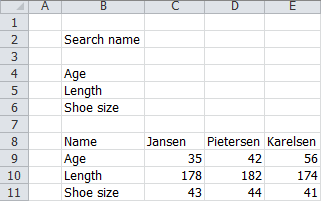Figure 6.27: Overview shoe sizes. Cell C2 is the input cell for the name to be searched. The cells C4:C6 are for the lookup formulas. File: Shoe_Sizes.xlsx 1. Open the practice file. 2. Select cell C4 and enter the formula =HLOOKUP($C$$2,$$C$$8:$$E$11,2,FALSE). As result, the error #N/A appears in the cell. This is because there is no search value in cell C2. 1. Enter in cell C2 the value Pietersen. In cell C4 now appears 42, the age. 2. Now enter the correct lookup formulas in the other two cells. • in C5 the formula: =HLOOKUP($C$$2,$$C$$8:$$E$11;3,FALSE). • in C6 the formula: =HLOOKUP($C$$2,$$C$$8:$$E$11;4,FALSE).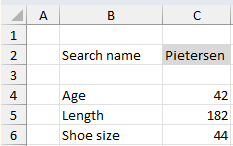Figure 6.28: Results HLOOKUP. ## 6.15 Exercises ### form001 - Results computer company The following table reflects the results of a computer company for the year 2010. The company’s target is to achieve a gross profit per product of more than$ 5000,- and a realized margin of more than 15%. Only if both targets are realized, the operating result can be characterized as good, in all other cases as bad.Create this model in a worksheet. Formulas should be used for the determination of the gross profit, the margin, and the result. If the gross profit and margin targets will be changed then the result should be adjusted.

File: Form001.xlsx

### form002 - Lookup article details

A computer store has the following article information in a table: article number, name, and price. To quickly search data from a specific article, the article number can be entered in a cell. The corresponding name and price are automatically looked up in the table.Enter the data in a worksheet and use formulas (VLOOKUP) to get the search results.

File: Form002.xlsx

### form003 - Calculating with dates

In the following table, you can enter your date of birth and the pension age (67 years). The date when you get your pension, the current date, and the number of days until your pension date should be calculated with formulas.Create this model in a worksheet and use formulas to calculate the output results.

File: Form003.xlsx

1. Used functions: DATE, YEAR, MONTH, DAY, TODAY.
2. A date layout like Wed 01/3/1967 can be get through the number format of the cell. Choose tab Home > Category Custom (group Number), or right-click on the cell and choose Format cells. In the box Type, you can enter the desired format. The format used in the example is ddd dd/m/yyyy. Try also other formats like dddd dd/mmm/yy to figure out how the formatting works.
3. A date in Excel is in reality just a number, with which you can make calculations. In order to determine the time difference between two dates, you can subtract these two dates.

A company with three salesmen has the objective that each salesman sells for at least $150,000 per year. If that amount is reached their commission will be 6.25% of that sales amount. If not, then their commission will be only 5.5%. In the following figure, you see a model to calculate the commission for each salesman.Create this model in a worksheet and use formulas for the calculation of the commissions. File: Form004.xlsx ### form005 - Birth dates A mother-child center in a hospital would like to have a daily overview of babies who are born too early, too late, and on time. For too early and too late they also want to see how many days too early or too late. And for babies who still have to be born, they want to see they are overdue.Create this model in a worksheet. Also, make a similar layout. File: Form005.xlsx 1. In the last four columns, the answer depends on one or more conditions, so you need to use the function IF. 2. In the case of two conditions, you can embed a second IF function within the first IF function. An alternative is to use the AND function within the IF function. 3. Birth date is too early when this date is before the expected date. 4. A date in Excel is in reality just a number, with which you can perform calculations. So you can determine whether a date is smaller, larger, or the same as another date. 5. If no date is known, then the appropriate field is empty. You can check in Excel if a field is empty. ### form006 - VAT return A company buys goods from suppliers and pays sales tax (VAT) to these suppliers. Then the company sells these goods to customers who have to pay VAT to the company. Every quarter, the company must submit a tax declaration. The difference between VAT received (through sales) and VAT paid (through purchases) must be paid to the tax authority. If this difference is negative, then there is a refund to the company. A small entrepreneur can get a reduction for the VAT through the so called Regulation Small Entrepreneurs (RSE). This regulation is worked out in the following table.1. Create a model with cells for data entry of the total sales and prepaid VAT and with cells for the constants (the thresholds and VAT percentage). Calculate in other cells the VAT initially to be paid, the reduction and the final amount to be paid or to received. Use only one VAT rate of 21%. The final amount should automatically be labeled as a payment or a receipt. All amounts must be formatted in whole euros. 2. Test the model thoroughly with all possibilities. File: Form006.xlsx ### form007 - Ticket price The following table shows the prices of a ticket for a sports game. There are two categories: children and adults. There is also a cell for entering the age (in whole years). After entering the age the ticket price is automatically calculated.Create this model in a worksheet. Use an IF function for the price calculation. Test the solution with different ages. File: Form007.xlsx ### form008 - Property tax The property tax has two parts, an owner part, and a user part. If the owner occupies the property himself, then he must pay both parts. The rate for both parts depends on the assessment of the value according to the WOZ act. The property tax for 2010 in a certain community amounts for the owner 0.1064% and for the user 0.1224% of the WOZ value.Create this model in a worksheet. The calculated values for owner and user depends on the answer “yes” or “no” on both questions. File: Form008.xlsx ### form009 - Savings deposit An amount of$ 20,000 will be put for a year in a savings deposit at an interest rate of 2.75%. Create a calculation model in which both the interest and the total amount after one year is calculated.

File: Form009.xlsx

Interest = $550.00 and Amount =$ 20,550.00

### form010 - Joule’s law

The amount of heat generated by an electric current flowing through a conductor can be calculated with Joule’s law: $$Q = 0.24*i^2*R*t$$

• Q: The amount of heat (cal)
• i: Current (ampere)
• R: Resistance (ohm)
• t: Time (sec)

Create a model in a worksheet in which current, the resistance, and the time can be entered and the amount of heat is calculated from the entered data.

File: Form010.xlsx

### form011 - Investment bond

A government bond with a maturity of 5 years annually pays a 3.5% interest rate. Suppose you want to have $300,000 over 5 years, how much do you need to invest in this bond? Use Excels function for present value (PV) to calculate the answer.$ 252,592

### form012 - Multiplication table

First, create the following table:Then enter in cell B2 a formula that you can copy down and to the right to the rest of the table so that the value inside each cell in the table is the multiplication of number before the row and the number above the column.

You will need a combination of absolute and relative addressing in your formula.

### form013 - Price olive oil

Olive oil can be purchased in cans of 5 liters. The price depends upon the number of cans:

• $45 per can for the first 20 cans •$40 for any can more than 20

Create a spreadsheet model where you can enter the total amount of cans and the total price should be calculated. Use cells for the price per can and the 20 cans limit. Give also names to all cells.